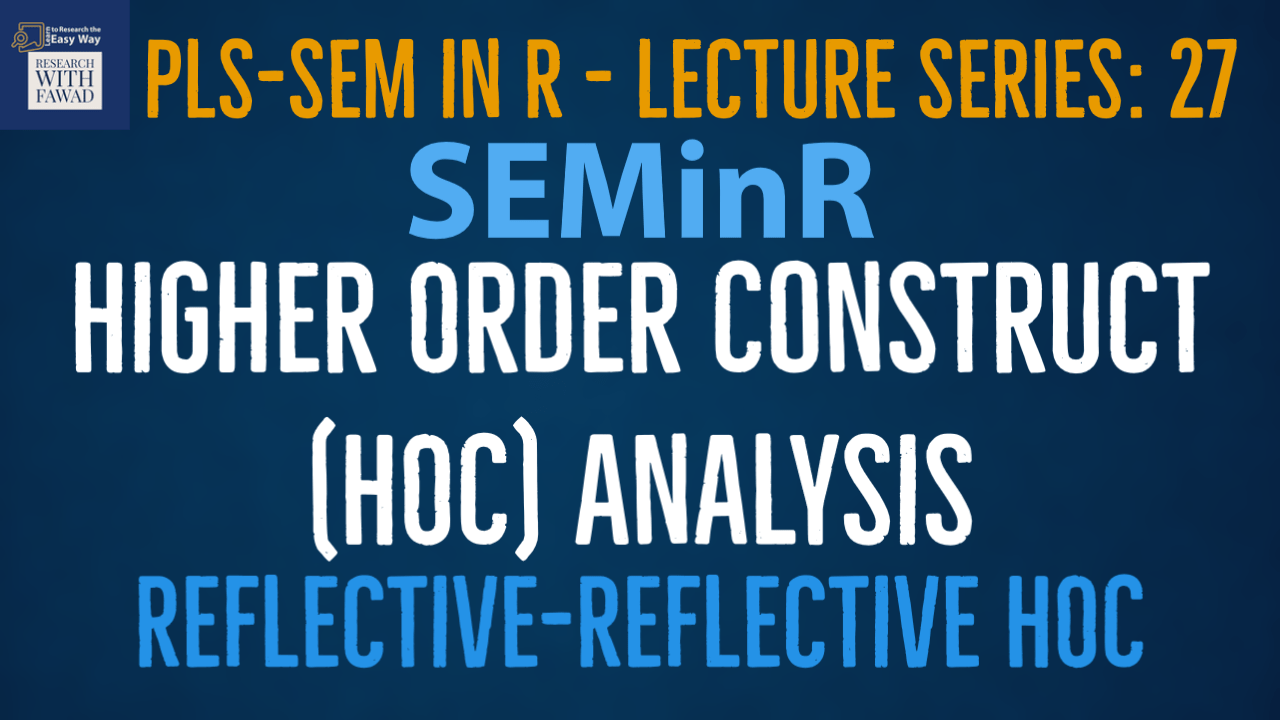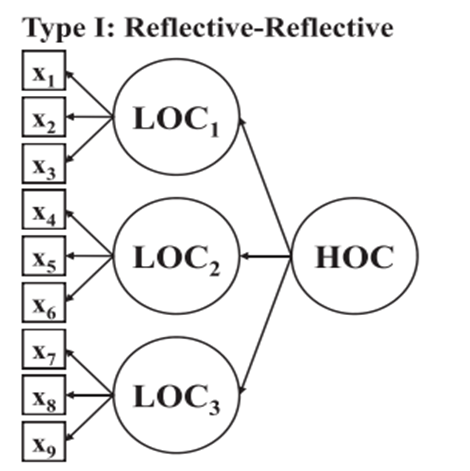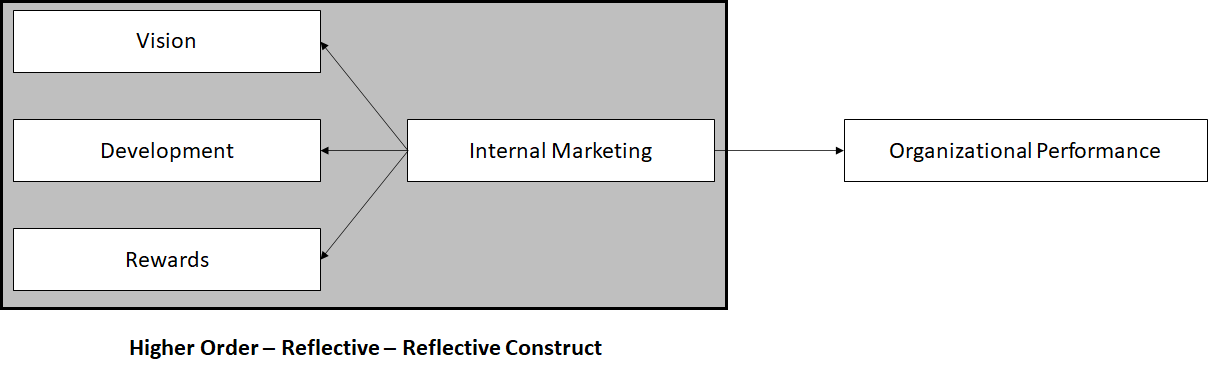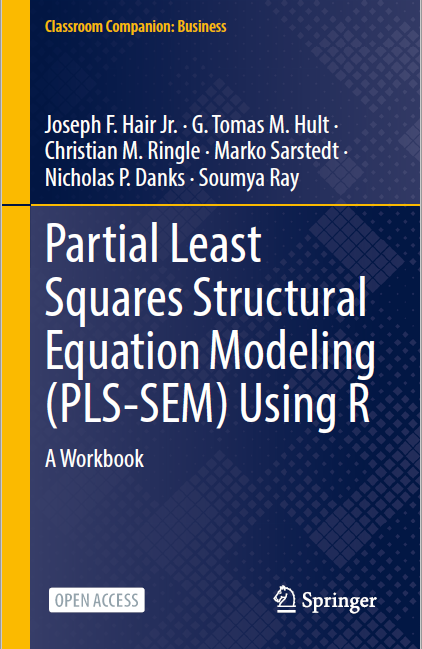# SEMinR Lecture Series - Higher Order Construct Analysis### SEMinR Lecture Series

This session is focused on validate and estimate Higher Order Constructs using SEMinR in Cran R

## Reflective-Reflective Higher ORder Construct

A widely debated Hierarchical Component Model (HCM) configuration is the reflective-reflective type. Critics argue that this type of HCM does not exist, or that it is meaningless because the reflective measures should be unidimensional and conceptually interchangeable. This conflicts with the view held by others that multiple underlying dimensions are distinct in nature.## Steps in Analyzing Reflective-Reflective Higher Order Construct

A Reflective-Reflective Higher Order model is assessed in two stages.

### Stage 1

• In the first stage create and estimate the model connecting all the lower-order components (Including Exogenous and Endogenous constructs). The model assessment first focuses on the reflective measurement models of the lower-order components. No Higher Order Constructs are modeled. Only LOCs for the HOCs are added to the estimation. In the first stage all the LOCs are assessed for Reliability and Validity. The Measurement model is assessed as we normally do. for further detail, Click Here
• After the Measurement model is assessed and reported (to learn how to report, Click here), use Latent Variable Score and save it in your data file to be used in the Second Stage.

### Stage 2

• In stage two, the latent variable scores of the lower-order components from stage one are used to create and estimate for the stage two model. (In SEMinR we do not need to do this manually as we have to in SmartPLS).
• The evaluation of stage two starts with focusing on the reflective measurement model of the higher-order component. For HOC, see the loadings of LOC for the HOC, assess the CR and AVE using the coefficients (loadings) enabling us to establish indicator reliabilities and AVE.
• These results above the critical value of 0.5. Cronbach’s alpha, CR and AVE establish reliability and convergent validity. Based on the HTMT criterion, discriminant validity with other LOCs can been established.
• Finally, the strcutural model is assessed for hypotheses testing.

Reference

Sarstedt, M., Hair Jr, J. F., Cheah, J. H., Becker, J. M., & Ringle, C. M. (2019). How to specify, estimate, and validate higher-order constructs in PLS-SEM. Australasian Marketing Journal (AMJ), 27(3), 197-211.

## Example

• To assess the impact of Internal Marketing on Organizational Performance.
• Internal Marketing is a higher order construct with 3 LOCs (Vision, Development, and Rewards).## Stage 1: SEMinR Code

``````library(seminr)
# Create measurement model
simple_mm = constructs(
composite("Vision", multi_items("VIS", 1:4)),
composite("Development", multi_items("DEV", 1:7)),
composite("Rewards", multi_items("RW", 1:4)),
composite("Organizational Performance", multi_items("OP", 1:5)))

# Create structural model
simple_sm = relationships(
paths(from = c("Vision", "Development", "Rewards"), to = "Organizational Performance"))

# Estimate the model
simple_model=estimate_pls(data=datas, measurement_model=simple_mm, structural_model=simple_sm)
plot(simple_model)

# Summarize the model results
summary_simple = summary(simple_model)
summary_simple\$reliability
summary_simple\$validity\$htmt
summary_simple\$validity\$fl_criteria``````

## Stage 2: SEMinR Code

``````library(seminr)
datas <- read.csv(file = "F:\\YouTube Videos\\SEMinR\\9. Evaluating Higher Order Model in SEMinR\\HOC in SEMinR\\Data.csv", header = TRUE, sep = ",")
# Create measurement model
simple_mm = constructs(
composite("Vision", multi_items("VIS", 1:4)),
composite("Development", multi_items("DEV", 1:7)),
composite("Rewards", multi_items("RW", 1:4)),
higher_composite("IM", c("Vision","Development","Rewards"), method = "two stage"),
composite("Organizational Performance", multi_items("OP", 1:5)))
# Create structural model
simple_sm = relationships(
paths(from = "IM", to = "Organizational Performance"))
# Estimate the model
simple_model=estimate_pls(data=datas, measurement_model=simple_mm, structural_model=simple_sm)
plot(simple_model)
# Summarize the model results
summary_simple = summary(simple_model)
summary_simple\$reliability
summary_simple\$validity\$htmt
summary_simple\$validity\$fl_criteria
# Bootstrap the model on the PLS Estimated Model
boot_model <- bootstrap_model(
seminr_model = simple_model,
nboot = 1000,
cores = parallel::detectCores(),
seed = 123)
# Store the summary of the bootstrapped model
# alpha sets the specified level for significance, i.e. 0.05
summary_boot <- summary(boot_model, alpha = 0.10)
summary_boot\$bootstrapped_paths
plot(boot_model)``````

## Reference

Hair Jr, J. F., Hult, G. T. M., Ringle, C. M., Sarstedt, M., Danks, N. P., & Ray, S. (2021). Partial Least Squares Structural Equation Modeling (PLS-SEM) Using R: A Workbook.The tutorials on SEMinR are based on the mentioned book. The book is open source and available for download under this link.